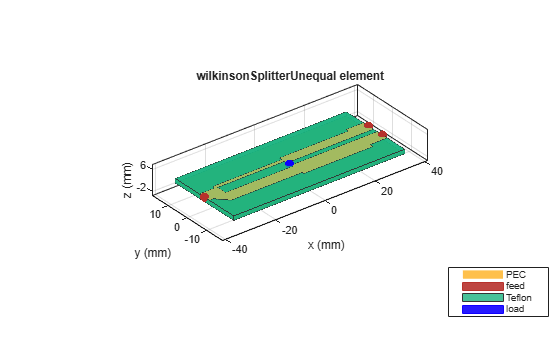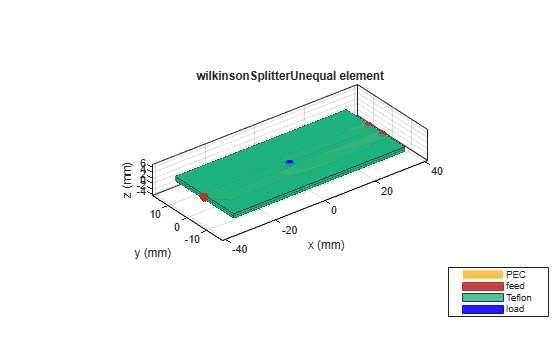# wilkinsonSplitterUnequal

Create unequal Wilkinson splitter

## Description

Use the `wilkinsonSplitterUnequal` object to create an unequal Wilkinson power splitter. You can use the unequal Wilkinson splitter to divide power unequally between two output ports. Unequal splitters are also used to feed power to antenna arrays for beam shaping.## Creation

### Syntax

``splitter = wilkinsonSplitterUnequal``
``splitter = wilkinsonSplitterUnequal(Name=Value)``

### Description

example

````splitter = wilkinsonSplitterUnequal` creates an unequal Wilkinson splitter with a Teflon substrate. The default properties are for a resonating frequency of 1 GHz.```

example

````splitter = wilkinsonSplitterUnequal(Name=Value)` sets Properties using one or more name-value arguments. For example, `wilkinsonSplitterUnequal(PortLineLength=0.0300)` creates a Wilkinson splitter with an input and output line length of 0.0300 meters. Properties not specified retain their default values.```

## Properties

expand all

Length of the input and the output line in meters, specified as a positive scalar.

Example: ```splitter = wilkinsonSplitterUnequal(PortLineLength=0.0070)```

Data Types: `double`

Width of the input and the output line in meters, specified as a positive scalar.

Example: ```splitter = wilkinsonSplitterUnequal(PortLineWidth=0.0070)```

Data Types: `double`

Length of the 70-ohm line in meters, specified as a positive scalar. The typical length of a Wilkinson splitter is λ/4.

Example: ```splitter = wilkinsonSplitterUnequal(SplitLineLength=0.0570)```

Data Types: `double`

Width of the 70-ohm line in meters, specified as a two-element vector of positive elements.

Example: ```splitter = wilkinsonSplitterUnequal(SplitLineWidth=[0.00780 0.00890])```

Data Types: `double`

Length of the output matching line in meters, specified as a positive scalar.

Example: ```splitter = wilkinsonSplitterUnequal(MatchLineLength=0.0780)```

Data Types: `double`

Width of the output matching line in meters, specified as a two-element vector of positive elements.

Example: ```splitter = wilkinsonSplitterUnequal(MatchLineWidth=[0.0049 0.0076])```

Data Types: `double`

Length of the resistor in meters, specified as a positive scalar.

Example: ```splitter = wilkinsonSplitterUnequal(ResistorSLength=0.0050)```

Data Types: `double`

Resistance value in ohms, specified as a positive scalar.

Example: `splitter = wilkinsonSplitterUnequal(Resistance=50)`

Data Types: `double`

Height of the Wilkinson splitter from the ground plane in meters, specified as a positive scalar.

Example: `splitter = wilkinsonSplitterUnequal(Height=0.0076)`

Data Types: `double`

Width of the ground plane in meters, specified as a positive scalar.

Example: ```splitter = wilkinsonSplitterUnequal(GroundPlaneWidth=0.046)```

Example: double

Type of dielectric material used as a substrate, specified as a `dielectric` object. The thickness of the default dielectric material Teflon is `0.0016` m or the same value as the `Height` property.

Example: `d = dielectric("FR4");` `splitter = wilkinsonSplitterUnequal(Substrate=d)`

Data Types: `string` | `char`

Type of metal used in the conducting layers, specified as a `metal` object.

Example: `m = metal("PEC");` `splitter = wilkinsonSplitterUnequal(Conductor=m)`

Data Types: `string` | `char`

## Object Functions

 `charge` Calculate and plot charge distribution `current` Calculate and plot current distribution `design` Design unequal Wilkinson splitter around specified frequency `feedCurrent` Calculate current at feed port `layout` Plot all metal layers and board shape `mesh` Change and view mesh properties of metal or dielectric in PCB component `shapes` Extract all metal layer shapes of PCB component `show` Display PCB component structure or PCB shape `sparameters` Calculate S-parameters for RF PCB objects

## Examples

collapse all

Create and view a default unequal Wilkinson splitter.

`splitter = wilkinsonSplitterUnequal`
```splitter = wilkinsonSplitterUnequal with properties: PortLineLength: 0.0070 PortLineWidth: 0.0051 SplitLineLength: 0.0279 SplitLineWidth: [0.0014 0.0049] MatchLineLength: 0.0277 MatchLineWidth: [0.0039 0.0066] ResistorLength: 0.0020 Resistance: 106 Height: 0.0016 GroundPlaneWidth: 0.0300 Substrate: [1x1 dielectric] Conductor: [1x1 metal] ```
`show(splitter)`Calculate and plot the S-parameters of the splitter at 3 GHz.

```spar=sparameters(splitter,3e9); figure rfplot(spar);```Create and view a multilayer unequal Wilkinson splitter.

```sub = dielectric(Name=["Teflon","Teflon"],EpsilonR=[2.1 2.1], ... LossTangent=[0 0],Thickness=[0.8e-3 0.8e-3]); unsplitter = wilkinsonSplitterUnequal(Height=0.8e-3,Substrate=sub); show(unsplitter)```Plot the charge and current on this splitter at 3 GHz.

```figure charge(unsplitter,3e9)``````figure current(unsplitter,3e9)```Pozar, David M. Microwave Engineering. 4th ed. Hoboken, NJ: Wiley, 2012.